Courses

# Test: Constructions- 1

## 25 Questions MCQ Test Mathematics (Maths) Class 10 | Test: Constructions- 1

Description
This mock test of Test: Constructions- 1 for Class 10 helps you for every Class 10 entrance exam. This contains 25 Multiple Choice Questions for Class 10 Test: Constructions- 1 (mcq) to study with solutions a complete question bank. The solved questions answers in this Test: Constructions- 1 quiz give you a good mix of easy questions and tough questions. Class 10 students definitely take this Test: Constructions- 1 exercise for a better result in the exam. You can find other Test: Constructions- 1 extra questions, long questions & short questions for Class 10 on EduRev as well by searching above.
QUESTION: 1

### To divide a line segment AB in the ratio 5 : 7, first a ray AX is drawn so that ∠BAX is an acute angle and then at equal distances points are marked on the ray AX such that the minimum number of these points is :

Solution: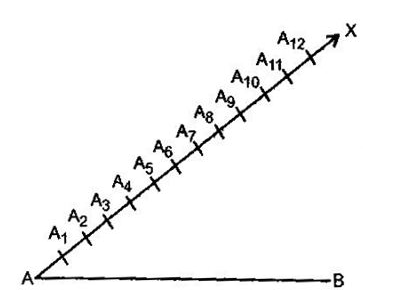According to the question, the minimum number of those points which are to be marked should be (Numerator + Denominator) i.e., 5 + 7 = 12

QUESTION: 2

### To divide a line segment AB in the ratio p : q ( p, q are positive integers), draw a ray AX so that ∠BAX s an acute angle and then mark points on ray AX at equal distances such that the minimum number of these points is :

Solution:

According to the question, the minimum number of those points which are to be marked should be (Numerator + Denominator) i.e., p + q

QUESTION: 3

### To construct a triangle similar to given ΔABC with its sides 8585 of the corresponding sides of ΔABC, draw a ray BX such that ∠CBX is an acute angle and X is one the opposite side of A with respect to BC. The minimum number of points to be located at equal distances on ray BX is :

Solution:

According to the question, the minimum number of points to be located at equal distances on ray BX is 8.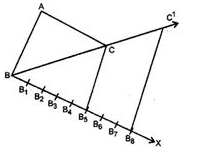QUESTION: 4

To divide a line segment AB in the ratio 5 : 6 draw a ray AX such that ∠BAX is an acute angel, then draw a ray BY parallel to AX and the points A_(1 ,) A_(2 ,) A_(3 ,) … and B_(1 ,) B_(2 ,) B_(3 ,)… are located a equal distances on ray AX and BY, respectively, Then the points joined are :

Solution:

The ratio is 5:6
Therefore, total number of divisons on BY and AX is 5+6=11.
ΔAOX and BOY are similar, since AX∥BY
⇒ ∠X = Y and ∠A = B
no. of divisions on B/no. of divisions on A = 6/5
number of divisions on B
⇒ number of divisions on B = 6/5× number of divisions in A
⇒ 6/5× 5 = 6
So, A5 should be joined to B6.

QUESTION: 5

To construct a triangle similar to given ΔABC with its sides 3/7 of the corresponding sides of ΔABC, first draw a ray BX such that ∠CBXis an acute angle and X lies on the opposite side of A with respect to BC. Then locate points B1,B2,B3, on BX equal distance and next step is to join :

Solution:

According to the question, the next step is to join is B7 to C.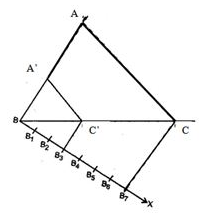QUESTION: 6

To divide a line segment AB in the ration 4 : 7, a ray AX is drawn first such that ∠BAX is an acute angle and then points A1,A2,A3,…are located at equal distances on the ray AX and the point B is joined to :

Solution:

According to question, the point B is joined to A11.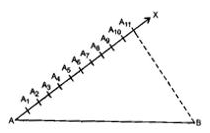QUESTION: 7

To divide a line segment AB in the ration 2 : 5, first a ray AX is drawn, so that ∠BAX is an acute angle and then at equal distances points are marked on the ray such that the minimum number of these points is :

Solution:

According to the question, the minimum number of those points which are to be marked should be (Numerator + Denominator) i.e. , 2 + 5 = 7

QUESTION: 8

To construct a triangle similar to given ΔABC with its sides 3/7 of the corresponding sides of ΔABC draw a ray BX such that ΔCBX is an acute angle and X is on the opposite side of A with respect to BC. The minimum number of points to be located at equal distances on ray BX is :

Solution:

According to question, the minimum number of points to be located at equal distances on ray BX is 7.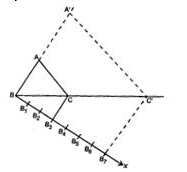QUESTION: 9

To divide a line segment PQ in the ratio 2 : 7, first a ray PZ is drawn so that ∠QPX is an acute angle and then at equal distances points are marked on the ray PX such that the minimum number of these points is :

Solution:

According to the question, the minimum number of those points which are to be marked should be (Numerator + Denominator) i.e., 2 + 7 = 9

QUESTION: 10

To divide a line segment LM in the ratio a : b, where a and b are positive integers, draw a ray LX so that ∠MLX is an acute angle and then mark points on the ray LX at equal distances such that the minimum number of these points is :

Solution:

According to the question, the minimum number of those points which are to be marked should be (Numerator + Denominator) i.e., a + b

QUESTION: 11

To construct a triangle similar to given ΔABC with its sides 7/4 of the corresponding sides of ΔABC, draw a ray BX such that ∠CBX is an acute angle and X is on the opposite side of A with respect to BC. The minimum number of points to be located at equal distances on ray BX is :

Solution:

When numerator is greater than denominator, then number of arcs should be drawnlarger of m and n. Therefore, according to question, the minimum number of points to be located at equal distances on ray BX is 7.

QUESTION: 12

To divide a line segment LM in the ratio 4 : 3, a ray LX is drawn firs such that ∠MLX is an acute angle and then points L1, L2, L3, … are located at equal distances on the ray LX and the points M is joined to :

Solution:

To divide a line segment AB in the ratio m : n, a ray AX is drawn in such that ∠BAX is an acute angle and then points A1, A2, A3, …….., Am, …….. An are located at equal distances on the ray AX and then the point B is joined to AnB.
• Therefore, according to question, the point M is joined to L7M.

QUESTION: 13

To construct a triangle similar to given ΔPQR with its sides 5/8 of the corresponding sides of ΔPQR, first a ray PXis drawn such that ∠QPX is an acute angle and X lies on the opposite side of R with respect to PQ. Then locate points P1, P2, P3…. OnPX at equal distances and next step is to join :

Solution:

To construct a triangle similar to given ΔABC with its sides mnmn of the corresponding sides of ΔABC, first a ray AX is drawn such that ∠BAX is an acute angle and X lies on the opposite side of C with respect to AB. Then locate points A1, A2, A3…. Am ….. An on AX at equal distances and next step is to join AnB. Therefore, according to question, the next step is to join P8 to Q.

QUESTION: 14

To divide a line segment AB in the ratio 3 : 7 , draw a ray AX such that ∠BAX is an acute angle, then draw a ray BY parallel to AX and the points A1,A2,A3, … and B1,B2,B3,… are located at equal distances on ray AX and BY respectively. Then the points joined are :

Solution:

If to divide the line segment AB in the ratio m : n, then we draw a ray AX such that ∠BAX is an acute angle, then draw a ray BY parallel to AX and the points A1,A2,A3, …….. Am-1, Am and B1,B2,B3, ….., Bn are located at equal distances on ray AX and BY respectively. Then the points joined are Am and Bn. Therefore, according to the question, the points joined are A3andB7.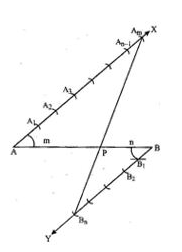QUESTION: 15

A divides the line segment PQ in the ratio :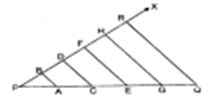Solution:

In the given figure, A divides the line segment PQ in the ratio 1 : 4.

QUESTION: 16

To draw a pair of tangents to a circle which are inclined to each other at an angle of 35°, it is required to draw tangents at the end-points of those two radii of the circle, the angle between which is

Solution:
QUESTION: 17

To divide a line segment PQ in the ratio 5 : 7, first a ray PX is drawn so that ∠QPX is an acute angle and then at equal distances points are marked on the ray PX such that the minimum number of these points is

Solution:
QUESTION: 18

The ____________ is the term used to denote a method in which case is driven into the ground and the material inside the casing is washed out and brought to the surface for inspection.

Solution:

The process of wash boring consists in driving an inner tube of diameter 25 mm to 50 mm, inside an outer tube of diameter 100 mm to 150 mm. Whereas, percussion boring and rotary drilling are the types of Deep boring.

QUESTION: 19

To divide a line segment AB internally in the 5 : 2, first a ray AX is drawn so that ∠BAX is an acute angle and then points A1,A2,A3.... are located at equal distance on ray AX and point B is joined to

Solution:

To divide a line segment AB in the ratio m : n (when m > n), a ray AX is drawn in such that ∠BAX is an acute angle and then points A1,A2,A3...Am,…..An…. are located at equal distances on the ray AX and then the point B is joined to AnB.
Therefore, according to the question, point B is joined toA7 .

QUESTION: 20

To divide a line segment AB internally in the ratio 4 : 7 , first a ray AX is drawn so that ∠BAX is an acute angle and then at equal distances points are marked on ray AX such that the minimum number of these points is

Solution:

According to the question, the minimum number of those points which are to be marked should be (Numerator + Denominator) i.e., 4 + 7 = 11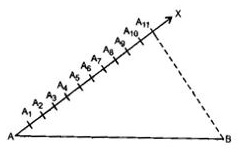QUESTION: 21

If the construction of a triangle ABC in which AB = 6 cm, ∠A = 70° and ∠B = 40° is possible then find the measure of ∠C.

Solution:
QUESTION: 22

To construct a triangle similar to a given triangle ABC with its sides 2/3 of the corresponding sides side of A with respect to BC. Then locate points X1,X2,X3… at equal distance on BX. The points to be joined in the next step are

Solution:

The points to be joined in the next step are Xand C.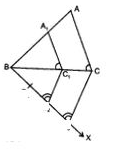QUESTION: 23

To construct a triangle similar to given ΔABC with its sides 7/5 of the corresponding side of ΔABC,draw a ray BX such that ∠CBX is an acute angle and X is on the opposite side of A with respect to BC. Then, locate points X1,X2,X3… at equal distances on BX. The points to be joined in the next step are

Solution:

According to the question, the points to be joined are Xand C.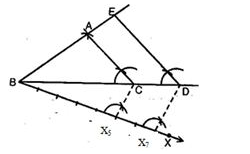QUESTION: 24

To divide line segment AB in the ration m : n (m, n are positive integers), draw a ray AX so that ∠BAX is an acute angle and then mark points on ray AX at equal distances such that the minimum number of these points is

Solution:

To divide line segment AB in the ration m : n (m, n are positive integers), draw a ray AX so that ∠BAX is an acute angle and then mark points on ray AX at equal distances such that the minimum number of these points is m + n.

QUESTION: 25

To divide a line segment AB in the ratio 5 :7, first a ray AX is drawn so that ∠BAX is an acute angle and then at equal distances, points are marked on the ray AX such that the minimum number of these points is :

Solution:

According to the question, the minimum number of those points which are to be marked should be (Numerator + Denominator) i.e., 5 + 7 = 12